# Foundations & Sorting on a Linear Array

Tom Kelliher, CS 315

Jan. 22, 1999

### From Last Time

1. Introduction.

2. No credit review quiz.

### Outline

1. Foundations: asymptotic analysis, mathematical induction, loop invariants.

2. Sorting on a linear array: linear arrays, sorting, time analysis

### Coming Up

Algorithm evaluation.

# Foundations

## Asymptotic Analysis

Define: O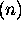,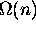,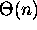, o, and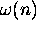, using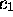,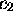, and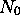.

Notes:

1. Throw out constants and lower order terms.

2. Meaning/use of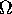and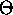.

## Mathematical Induction

Prove that a complete binary tree of height h has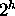leaves.

Basis, inductive hypothesis, inductive step.

## Loop Invariants

Four characteristics:

1. Must be true upon entry into the loop
2. Execution of the loop body must preserve the invariant
3. Invariant must capture the correctness and the meaning of the loop
4. Loop must terminate

Consider the following sorting algorithm which sorts the n elements of data (the elements are indexed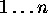):

```      for i := n downto 2 do
largest := i;

for j := i-1 downto 1 do
if data[j] > data[largest]
largest := j;

swap(data[largest], data[i]);
```

Prove that the algorithm sorts the elements of the array data into ascending order.

First, demonstrate the algorithm on the array

```int data[] = { 12, 19, 6, 15 };
```

# Sorting on a Linear Array

## Linear Arrays

A linear array: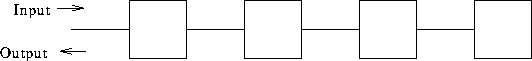Cell properties:

1. Local program: a ``few'' operations.

2. Local storage: a ``few'' words of data.

3. Operation sequence:

3. Perform computation indicated by local program.

4. Generate output for neighbors.

5. Update local storage.

4. Synchronized to a global clock.

5. Must all the programs be identical? Elegance?

Communication properties:

2. Left, right neighbors.

3. Fixed connection network.

4. Data pulsing through network: systolic computation.

## Sorting

1. Phase 1: sorting program.
1. Accept input from left neighbor.

2. Compare to stored value.

3. Output larger value to right neighbor.

4. Store smaller value.

2. Phase 2: output. Begin passing left when no more input is received from the left neighbor.

Example: sort 12, 19, 6, 15.

How many steps to sort? How many steps to output?

Thomas P. Kelliher
Fri Jan 22 09:23:26 EST 1999
Tom Kelliher